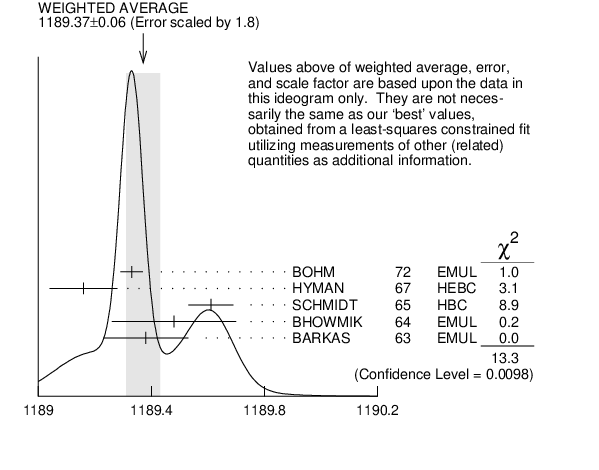# ${{\boldsymbol \Sigma}^{+}}$ MASS INSPIRE search

The fit uses ${{\mathit \Sigma}^{+}}$, ${{\mathit \Sigma}^{0}}$, ${{\mathit \Sigma}^{-}}$, and ${{\mathit \Lambda}}$ mass and mass-difference measurements.
VALUE (MeV) EVTS DOCUMENT ID TECN  COMMENT
$\bf{ 1189.37 \pm0.07}$ OUR FIT  Error includes scale factor of 2.2.
$\bf{ 1189.37 \pm0.06}$ OUR AVERAGE  Error includes scale factor of 1.8.
$1189.33$ $\pm0.04$ 607 1
 1972
EMUL
$1189.16$ $\pm0.12$
 1967
HEBC
$1189.61$ $\pm0.08$ 4205
 1965
HBC See note with ${{\mathit \Lambda}}$ mass
$1189.48$ $\pm0.22$ 58 2
 1964
EMUL
$1189.38$ $\pm0.15$ 144 2
 1963
EMUL
1  BOHM 1972 is updated with our 1973 ${{\mathit K}^{-}}$, ${{\mathit \pi}^{-}}$, and ${{\mathit \pi}^{0}}$ masses (Reviews of Modern Physics 45 S1 (1973)).
2  These masses have been raised 30 keV to take into account a 46 keV increase in the proton mass and a 21 keV decrease in the ${{\mathit \pi}^{0}}$ mass (note added 1967 edition, Reviews of Modern Physics 39 1 (1967)).${{\mathit \Sigma}^{+}}$ mass (MeV)
References:
 BOHM 1972
NP B48 1 Determination of the Masses of the Charged ${{\mathit \Sigma}}$ Hyperons
 HYMAN 1967
PL 25B 376 Mass Measurements Using the Range Energy Relation in a Helium Bubble Chamber
 SCHMIDT 1965
PR 140 B1328 Precise Hyperon Masses
 BHOWMIK 1964
NP 53 22 The Lifetimes of Charged ${{\mathit \Sigma}}$ Hyperons
 BARKAS 1963
PRL 11 26 Resolution of the ${{\mathit \Sigma}^{-}}$-Mass Anomaly